Related Articles
random header | Set 2 (Distributions)
• Difficulty Level : Easy
• Last Updated : 29 May, 2017

Set 1 (Generators)

Distributions

1. uniform_int_distribution: It produces random integer values i, which are uniformly distributed on the closed interval [a,b], which is described by the following probability mass function: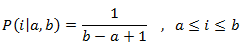• operator(): It generates the random number that are distributed according to the probability function.
• min: It returns the greatest lower bound of the range of values returned by operator(), which is the distribution parameter ‘a’ for uniform_int_distribution.
• max: It returns the least upper bound of the range of values returned by operator(), which is the distribution parameter ‘b’ for uniform_int_distribution.
• reset: It resets the distribution, so that on the subsequent uses the result does not depends on the values already produced by it.
 `// C++ program to illustrate``// the use of operator()``// in uniform_int_distribution``#include ``#include ``using` `namespace` `std;`` ` `// Driver program``int` `main()``{``     ` `    ``// Constructing a trivial random generator engine ``    ``unsigned s = 2;``     ` `    ``// The random number generator``    ``default_random_engine generator (s);``     ` `    ``uniform_int_distribution<``int``> distribution(1,10);``    ``cout << ``"Some random numbers between 1 and 10"``;``    ``for` `(``int` `i = 0; i < 10; ++i)``        ``cout << distribution(generator) ;``     ` `    ``cout << endl;``     ` `    ``return` `0;``}`

Output:

```Some random numbers between 1 and 10: 1 3 6 10 1 5 1 4 4 9
```
 `// C++ program to illustrate``// the use of reset``// in uniform_int_distribution``#include ``#include ``using` `namespace` `std;`` ` `//Driver program``int` `main()``{``         ` `    ``//the random number generator``    ``default_random_engine generator;``     ` `    ``// Initialising the uniform distribution``    ``uniform_int_distribution<``int``> distribution(1, 1000);``     ` `    ``// First random number is generated``    ``cout << distribution(generator) << endl;``     ` `    ``//Resets the distribution``    ``distribution.reset();``     ` `    ``// Second random number is ``    ``//generated independent of previous number``    ``cout << distribution(generator) << endl;``     ` `    ``return` `0;``}`

Output:

```1
132
```
2. uniform_real_distribution: It is the random number distribution that produces floating-point values , which is described by the following probability density function: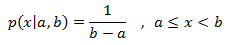• operator(): It returns a new random number that follows the distribution’s parameters.
• min: It returns the greatest lower bound of the range of values returned by operator(), which is the distribution parameter ‘a’ for uniform_real_distribution.
• max: It returns the least upper bound of the range of values returned by operator(), which is the distribution parameter ‘b’ for uniform_real_distribution.
• reset: It resets the distribution, so that on the subsequent uses the result does not depend on values already produced by it.
 `// C++ program to illustrate``// the use of operator()``// in uniform_int_distribution``#include ``#include ``using` `namespace` `std;`` ` `// Driver program``int` `main()``{``     ` `    ``// Constructing a trivial random generator engine ``    ``unsigned s = 2;``     ` `    ``// The random number generator``    ``default_random_engine generator (s);``     ` `    ``uniform_int_distribution<``int``> distribution(1,10);``    ``cout << ``"Random numbers between 1 and 10"``;``    ``for` `(``int` `i = 0; i< 10; ++i)``        ``cout << distribution(generator) ;``     ` `    ``cout << endl;``     ` `    ``return` `0;``}`

Output:

```some random numbers between 0.0 and 10.0:
0.150031
9.77072
3.36669
7.06447
5.11455
8.43061
1.93792
7.78965
8.31532
5.14354
```
 `// C++ program to illustrate``// the use of reset``// in uniform_real_distribution``#include ``#include ``using` `namespace` `std;`` ` `// Driver program``int` `main()``{``    ``default_random_engine generator;``    ``uniform_real_distribution<``double``> distribution(0.0,100.0);``     ` `    ``// It prints two independent values:``    ``// First random number is generated``    ``cout << distribution(generator) << endl;``     ` `    ``//Resets the distribution``    ``distribution.reset();``     ` `    ``// Second random number is ``    ``//generated independent of previous number``    ``cout << distribution(generator) << endl;``     ` `    ``return` `0;``}`

Output:

```13.1538
45.865
```

II. Related to bernoulli trials: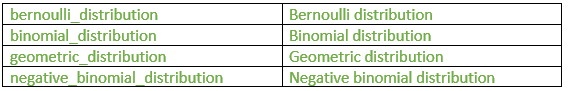1. bernoulli_distribution: It is the random number distribution that produces bool values according to a Bernoulli distribution, given by the following probability mass function:• operator(): It returns a new random number.
• min: It returns the greatest lower bound of the range of values returned by operator(), which for bernoulli_distribution is false.
• max: It returns the least upper bound of the range of values returned by operator(), which for bernoulli_distribution is true.
 `// C++ program to illustrate``// the bernoulli_distribution``#include ``#include ``using` `namespace` `std;`` ` `//Driver program``int` `main()``{``    ``const` `int` `temp=500;``     ` `    ``//The random number generator``    ``default_random_engine generator;``     ` `    ``//Initialising the bernoulli distribution``    ``bernoulli_distribution distribution(0.7);``     ` `    ``// count number of trues``    ``int` `count=0; ``     ` `    ``for` `(``int` `i = 0; i < temp; ++i) ``    ``{``         ` `        ``// checking for true condition ``        ``if` `(distribution(generator)) ``        ``count++;``    ``}``     ` `    ``cout << ``"bernoulli_distribution (0.7) x 500:"` `<< endl;``    ``cout << ``"true: "` `<< count << endl;``    ``cout << ``"false: "` `<< temp-count << endl;``     ` `    ``return` `0;``}`

Output:

```bernoulli_distribution (0.7) x 500:
true:  360
false: 140
```
 `// C++ program to``// illustrate the use of reset``#include ``#include ``using` `namespace` `std;`` ` `//Driver program``int` `main()``{``    ``// Random number generator``    ``default_random_engine generator;``     ` `    ``// Initialising the bernoulli distribution``    ``bernoulli_distribution distribution;``     ` `    ``// print two independent values:``    ``cout << distribution(generator) << endl;``     ` `    ``// use of reset``    ``// Generates second output without``    ``// the effect of first output ``    ``distribution.reset();``    ``cout << distribution(generator) << endl;`` ` `return` `0;``}`

Output:

```1
1
```
2. binomial_distribution: It is the random number distribution that produces integers according to a binomial discrete distribution, which is given by this probability mass function:• operator(): It generates a new random number.
• max: It returns the least upper bound of the range given by operator(), which for binomial_distribution is the distribution parameter t.
• min: It returns the greatest lower bound of the range given by member operator(), which for binomial_distribution is always zero.
• reset: It resets the distribution, so that subsequent uses of the object do not depend on values already produced by it.
 `// C++ program to illustrate``// the use of binomial_distribution``#include ``#include ``#include ``using` `namespace` `std;`` ` `int` `main()``{``     ` `    ``// construct a trivial random ``    ``//generator engine from a time-based seed:``    ``unsigned seed = chrono::system_clock::now().time_since_epoch().count();``    ``default_random_engine generator (seed);``     ` `    ``// Initialising binomial distribution``    ``binomial_distribution<``int``> distribution (15, 0.4);``     ` `    ``cout << ``"some binomial results (t=15, p=0.4): "``;``    ``for` `(``int` `i = 0; i < 15; ++i)``    ``{``             ` `        ``// Use of operator()``        ``cout << distribution(generator) << ``" "``;``    ``}``    ``cout << endl;``     ` `    ``return` `0;``}`

Output:

```some binomial results (t=15, p=0.4): 7 6 7 8 4 6 7 6 9 3 5 6 4 6 7
```
 `// C++ program to illustrate``// the use of binomial_distribution``#include ``#include ``#include ``using` `namespace` `std;`` ` `int` `main()``{``     ` `    ``// construct a trivial random ``    ``//generator engine from a time-based seed:``    ``unsigned seed = chrono::system_clock::now().time_since_epoch().count();``    ``default_random_engine generator (seed);``     ` `    ``// Initialising binomial distribution``    ``binomial_distribution<``int``> distribution (15, 0.4);``     ` `    ``cout << ``"some binomial results (t=15, p=0.4): "``;``    ``for` `(``int` `i = 0; i < 15; ++i)``    ``{``             ` `        ``// Use of operator()``        ``cout << distribution(generator) << ``" "``;``    ``}``    ``cout << endl;``     ` `    ``return` `0;``}`

Output:

```57
52
```
3. geometric_distribution: It is a random number distribution that produces integers according to a geometric discrete distribution, given by the following probability mass function:• operator(): It returns a new random number that follows the distribution’s parameters.
• max: It returns least upper bound of the range given by operator().
• min: It returns the minimum value given by operator().
• reset: It resets the distribution, so that subsequent uses of the object do not depend on values already produced by it.
 `// C++ program to illustrate``//the use of geometric_distribution ``#include ``#include ``#include ``#include ``using` `namespace` `std;`` ` `int` `main()``{``    ``// construct a trivial random``    ``// generator engine from a time-based seed:``    ``int` `seed = chrono::system_clock::now().time_since_epoch().count();``    ``default_random_engine generator (seed);``     ` `    ``// Initialises the geometric distribution``    ``geometric_distribution<``int``> distribution (1.0 / 5);``     ` `    ``cout << ``"Plus sign is 5 spaces away from the next :"` `<< endl;``    ``for` `(``int` `i = 0; i < 10 ; ++i) ``    ``{``        ``int` `number = distribution(generator);``        ``cout << string (number,``' '``) << ``"+"``;``    ``}`` ` `return` `0;``}`

Output:

```each plus sign is 5 spaces away from the next :
++ + +   +  ++     +        ++
```
 `// C++ program to illustrate ``// the use of reset``#include ``#include ``using` `namespace` `std;`` ` `// Driver program``int` `main()``{``     ` `    ``// Random number generator ``    ``default_random_engine generator;``     ` `    ``// Initialising the geometric distribution``    ``geometric_distribution<``int``> distribution(0.3);``     ` `    ``// Prints two independent values:``    ``// Generates the first value``    ``cout << distribution(generator) << endl;``     ` `    ``// Use of reset``    ``distribution.reset();``     ` `    ``// Generates second value``    ``cout << distribution(generator) << endl;``     ` `    ``return` `0;``}`

Output:

```0
1
```
4. negative_binomial_distribution: It is a random number distribution that produces integers according to a negative binomial discrete distribution (also known as Pascal distribution), given by the following probability mass function: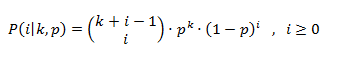• operator():It returns a new random number which follows the distribution’s parameters.
• max:It returns least upper bound of the range given by operator().
• min:It returns the minimum value given by operator(),which for negative_binomial_distribution is always zero.
• reset: It resets the distribution, so that subsequent uses of the object do not depend on values already produced by it.
 `// C++ program to illustrate``// the use of operator() in``// negative_binomial_distribution ``#include ``#include ``#include ``using` `namespace` `std;`` ` `// Driver program``int` `main()``{``    ``// construct a trivial random ``    ``// generator engine from a time-based seed:``    ``unsigned seed = chrono::system_clock::now().time_since_epoch().count();``    ``default_random_engine generator (seed);``     ` `    ``// Initialising negative binomial distribution``    ``negative_binomial_distribution<``int``> distribution (6,0.7);``     ` `    ``cout << ``"Negative binomial results (t=6, p=0.7): "``;``    ``for` `(``int` `i = 0; i < 15; ++i)``        ``{``            ``// Use of operator``            ``cout << distribution(generator) << ``" "``;``        ``}``     ` `    ``cout << endl;``     ` `    ``return` `0;``}`

Output:

```Negative binomial results (t=6, p=0.7): 2 6 3 1 4 1 4 1 2 0 7 3 4 4 4
```
 `// C++ program to illustrate``// the use of reset in``// negative_binomial_distribution::``#include ``#include ``using` `namespace` `std;`` ` `// Driver program``int` `main()``{``     ` `    ``// Random number generator ``    ``default_random_engine generator;``     ` `    ``// Initialising the negative binomial distribution``    ``negative_binomial_distribution<``int``> distribution(20, 0.5);``     ` `    ``// print two independent values:``    ``// Generates the first value``    ``cout << distribution(generator) << endl;``     ` `    ``// Use of reset``    ``distribution.reset();``     ` `    ``// Generates the second value``    ``cout << distribution(generator) << endl;``     ` `    ``return` `0;``}`

Output:

```23
30
```

III.Piece wise distributions:1. discrete_distribution: It is a random number distribution that produces integer values according to a discrete distribution.
• operator(): It returns a new random number that follows the distribution’s parameters.
• max: It returns the least upper bound of the range given by operator().
• min: It returns the greatest lower bound of the range given by operator().
• reset: It resets the distribution, so that subsequent uses of the object do not depend on values already produced by it.
 `// C++ program to illustrate the``// use of operator() in``// discrete_distribution``#include ``#include ``using` `namespace` `std;`` ` `int` `main()``{``     ` `    ``// number of experiments``    ``int` `n = 10000; ``     ` `    ``// maximum number of stars to distribute``    ``int` `m = 100; ``     ` `    ``// Random number generator``    ``default_random_engine generator;``     ` `    ``//Initialising discrete distribution``    ``discrete_distribution<``int``> distribution { 2, 2, 1, 1, 2, 2, 1, 1, 2, 2 };``     ` `    ``int` `p = {};``     ` `    ``// use of operator()``    ``for` `(``int` `i = 0; i < n; i++) ``    ``{``        ``int` `number = distribution(generator);``        ``p[number]++;``    ``}``     ` `    ``cout << ``"a discrete_distribution:"` `<< endl;``    ``for` `(``int` `i = 0; i < 10; ++i)``    ``{``        ``cout << i << ``": "` `<< string(p[i]*m/n,``'*'``) << endl;``    ``}``     ` `    ``return` `0;``}`

Output:

```a discrete_distribution:
0: ************
1: *************
2: *****
3: ******
4: ************
5: ************
6: ******
7: ******
8: ************
9: ************
```
 `// C++ program to illustrate``//the use of reset in``//discrete_distribution``#include ``#include ``using` `namespace` `std;`` ` `// Driver program``int` `main()``{``     ` `    ``// Random number generator ``    ``default_random_engine generator;``     ` `    ``// Initialising the discrete distribution``    ``discrete_distribution<``int``> distribution {20,20,30,40};``     ` `    ``// print two independent values:``    ``// Generates the first value``    ``cout << distribution(generator) << endl;``     ` `    ``// Use of reset``    ``distribution.reset();``     ` `    ``// Generates the secong value``    ``cout << distribution(generator) << endl;``     ` `    ``return` `0;``}`

Output:

```0
2
```
2. piecewise_constant_distribution: It is a random number distribution that produces floating-point values that are uniformly distributed over each of a sequence of contiguous subintervals, given by following probability density function: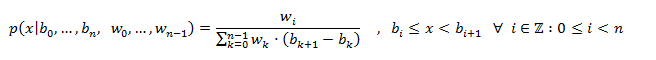• operator(): It returns a new random number that follows the distribution’s parameters.
• max: It returns the least upper bound of the range given by operator().
• min: It returns the greatest lower bound of the range given by operator().
• reset: It resets the distribution, so that subsequent uses of the object do not depend on values already produced by it.
 `// C++ program to illustrate the``// use of reset in``// piecewise_constant_distribution``#include ``#include ``using` `namespace` `std;`` ` `// Driver program``int` `main()``{``     ` `    ``// Random number generator``    ``default_random_engine generator;``     ` `    ``// Initialisind piecewise_constant_distribution``    ``piecewise_constant_distribution<``double``> distribution ``                ``( 4, 0.0, 10.0, [](``double` `x){``return` `x;} );``     ` `    ``// print two independent values:``    ``// Generates the first value``    ``// Use of operator()``    ``cout << distribution(generator) << endl;``     ` `    ``// Use of reset``    ``distribution.reset();``     ` `    ``// Generates second value``    ``cout << distribution(generator) << endl;``     ` `    ``return` `0;``}`

Output:

```3.4205
6.6692
```
3. piecewise_linear_distribution: It is a random number distribution that produces floating-point values that are distributed over a sequence of contiguous subintervals.
• operator():It returns a new random number that follows the distribution’s parameters.
• max: It returns the least upper bound of the range given by operator().
• min: It returns the greatest lower bound of the range given by operator().
• reset: It resets the distribution, so that subsequent uses of the object do not depend on values already produced by it.
 `// C++ program to illustrate the``// use of reset in``// piecewise_linear_distribution``#include ``#include ``using` `namespace` `std;`` ` `// Driver program``int` `main()``{``     ` `    ``// Random number generator``    ``default_random_engine generator;``     ` `    ``// Initialising piecewise_linear_distribution``    ``piecewise_linear_distribution<``double``>``        ``distribution ( 5, 0.0, 10.0, [](``double` `x){``return` `x+1.0;} );``     ` `    ``// print two independent values:``    ``// generates first value``    ``// use of operator()``    ``cout << distribution(generator) << endl;``     ` `    ``// Use of reset``    ``distribution.reset();``     ` `    ``// generates second value``    ``cout << distribution(generator) << endl;``     ` `    ``return` `0;``}`

Output:

```2.48143
6.07656
```

This article is contributed by Shambhavi Singh. If you like GeeksforGeeks and would like to contribute, you can also write an article using contribute.geeksforgeeks.org or mail your article to contribute@geeksforgeeks.org. See your article appearing on the GeeksforGeeks main page and help other Geeks.

Please write comments if you find anything incorrect, or you want to share more information about the topic discussed above.

Want to learn from the best curated videos and practice problems, check out the C++ Foundation Course for Basic to Advanced C++ and C++ STL Course for foundation plus STL.  To complete your preparation from learning a language to DS Algo and many more,  please refer Complete Interview Preparation Course.
My Personal Notes arrow_drop_up